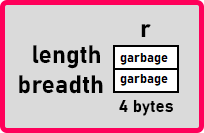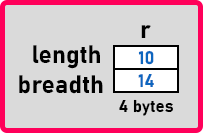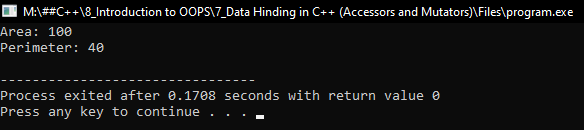# Data Hiding in C++

## Data Hiding in C++ with Examples

In this article, I am going to discuss Data Hiding in C++ with Examples. Please read our previous article where we discussed How to Create Objects in Heap Memory using C++ with Examples. At the end of this article, you will learn the objective of data hiding and why do you want to hide data.

##### Data Hiding in C++:

Let us learn about data hiding. This is one of the important topics in object orientation and data hiding is related to encapsulation. After encapsulation, we can also achieve data hiding. What does it mean? Let us see.

```class Rectangle
{
public:
int length;
int Area ()
{
}
int Perimeter ()
{
return 2 * (length + breadth);
}
};```

A class will contain data and functions. So, data members and member functions are together here inside this class. So by writing everything inside a block i.e. ‘{ }’ we are achieving encapsulation. But the problem here is we have made everything as public, data as well as functions.

In our previous articles already we have given some examples that if you see a television box, everything is kept inside the box and even there will be a warranty seal on the box and what is visible outside? the buttons that are nothing but functions. Press that button then the function will be performed. Volume change, volume will increase or decrease like that. For all these operations buttons are available. So those buttons are nothing but functions. And when you press the button, something happens inside the circuit board to respond to that particular function.

Similarly, if you take an example of a car everything is covered inside the bonnet and nothing is visible. Only the functions are visible that are at the dashboard and whatever the operation that you perform affects the engine that is inside the bonnet. But you cannot access bonnet directly. You can access everything through functions that are acceleration and change of gear and all these things.

By observing the products of other engineering, we can find that only the functions should be made public, and data should be made private because if the data is public, we are able to directly create the object and access data.

Imagine it. we have a television box and there are some wires coming out of the box. The wires that are hanging out, now you can join those wires. Some functions will be performed if you join the wrong wires then the television may not work properly.

Suppose a television belongs to your company. I have purchased this television and I’m playing with those wires. If something wrong happens then who do I blame? Your company. You did not make this television properly. I was joining the wires only but the television is not working properly, so you will be blamed. You should not have anything coming out of the television. For all the functions you provide buttons but if buttons are not working then you are responsible. Because what is happening inside, I don’t know. I am a user. So, you should provide functions and when I call those functions if it is not working properly then you are responsible for that.

int main(){
Rectangle r;
r.length = 10;
}

In the same way, here we are directly accessing variables of the class or data members of the class. If this is your class and if anything goes wrong then I will blame you. If you hide everything and say you can access everything through a function then you can control everything.

We have discussed so many things related to data hiding and we have given various examples. Now let us see how these things are related. As in the ‘Rectangle’ class, we made data members public then if I write,

r.length = -5;

Can length be negative? I don’t know. I’m just a user. I’m concentrating on my main function. I don’t know what is ‘Rectangle’ class and I don’t even know that length and breadth can be negative. Now if we write,

cout << r.Area() << endl;

What will be the output? -50. The area cannot be negative. The area is negative because we have set a negative length. So, I’m mishandling things. The things are going wrong because you have kept length and breadth public and you are allowing me to access them. So, I can say that your class is wrong. Your class is not perfect because it is giving negative areas.

So, this is the philosophy behind object-orientation. If you think in this way, then you can design classes properly. So that data members should not be made public.

##### Why data members should not be made public?

If data members are made public then there are chances that they may be mishandled. If mishandling is done the functions of a class may not give the correct results and we cannot rely on such classes, though it is a mistake of a programmer. But the class will also be blamed equally for giving wrong results.

##### How to Achieve Data Hiding in C++ (Accessors and Mutators)?

We will make the data members private and member functions public,

```class Rectangle
{
private:
int length;
public:
int Area ()
{
}
int Perimeter ()
{
return 2 * (length + breadth);
}
};```

From the point where we have written public, everything below that becomes public. And same with private. Here we have made data members private. And by default, everything is private in a class. We can skip writing private for data members as by default they are private.

##### Can we access length or breadth now?

No, we cannot write the value of length or breadth and even we cannot read the value of these variables. Then how we can set length and breadth? We cannot set it directly. If we write,

Rectangle r;
cout << r.Area() << endl;

Now, what result we will get? See the object ‘r’ is having its length and breadthWe have not initialized the variables as we are not allowed to initialize them. So, both the variables will have some garbage value. Then if we print the area, the area function will multiply these two garbage values and give some garbage value. This is the problem. We are unable to set that length and breadth then how to set these values? As we said that function should be public so there should be a ‘set’ function for setting the length and breadth. And also, when we want to read it there should be a ‘get’ function for getting the value of length and breadth. So, let’s write those functions.

For setting length, we have,
void setLength(int l){
length = l;
}
This is the function for setting the length of the rectangle. This is taking one parameter which is the length that we want to set. And inside this, we assigned the value of ‘l’ to the length variable. For setting breadth, we have,
}
Here we assigned the value of ‘b’ to the breadth variable. So, these are the setters that will set the given value of length and breadth. Now let us write getters.
int getLength(){
return length;
}
}
These are the getter functions for length and breadth. Both the functions are of type ‘int’ as they are returning an integer value. Now how to use these functions? Let us see.
int main(){
Rectangle r;
r.setLength(10);
}
Here we have an object of type rectangle in our main function. Then we have called the ‘setLength’ and ‘setBreadth’ functions and passed some values as parameters. ‘10’ will store in length and ‘14’ will store in breadth.We have not directly set the value of length and breadth but we are calling the functions of a class. So, this is a better way. You can call the functions and set the values. So, it is a good practice but still, the problem is as it is. See if we say

It will take -5 and set it into the breadth and still breadth is -5 again. Then what is the benefit of writing the functions? Now we will make those functions a little smart by checking the data they are getting and then setting it. Let us modify those functions.

void setLength(int l){
if(l >= 0)
length = l;
else
length = 0;
}
if(b >= 0)
else
}

Now the setLength function is validating the data that it is getting. If ‘l’ is greater than 0 then the only length will be assigned to the length variable otherwise length will be 0. Same with breadth function. We can also write a statement inside setLength or setBreadth function that will inform us that the data is invalid or negative. Now we have a setLength and setBreadth functions which validate the data and assign it. So, if we write,

int main(){
Rectangle r;
r.setLength(10);
cout << r.Area() << endl;
}

Here we are passing -5, so the breadth function will set the breadth as zero. Next, we have called the area function. Then what will we get? See length is 10 and breadth is 0 then the area will be 0. By using the function, we have created some conditions that should be followed to assign values to data members. With this, we will not get any wrong results or negative results. But still, the main function is not knowing or the user is not knowing that there is a problem with the negative value. So, if you want you can write “length or breadth cannot be negative” inside the setLength and setBreadth function. Next, we want to display the length of a rectangle or breadth of a rectangle. So, we can say,

r.getLength();

It will give the value of Length and Breadth and print on the screen. Finally, we have achieved data hiding. We made the data private and made the functions public and because we were unable to access the data, we have provided some validating functions or intelligence which will take the valid data and assign it to those data members. If it is invalid then you can print a message that tells the user that the data is invalid.

##### How many functions we have written?

The setLengh, setBreadth, getLength and getbreadth. We have written two data functions get and set. The ‘get’ will give you the value of data members and the ‘set’ will change the value of the data members. So, whatever the data member, if it is length then it would getLength, if it is breadth then it will getBreadth, if it is marks then getMarks, if it is prize then getPrize. These functions are called Accessors and all the set functions are called Mutators. Both the functions setters and getters are known as property functions. Because data numbers are called property. So, these types of functions are called property functions, and the one which is reading the value is called accessors and the one which is writing the value is called mutator.

Now few more things, for any data member that is length or breadth, if you are writing both get and set function then the property is a read writeable. If you write only accessors or get functions and don’t write a set function, then it is read-only. You cannot write the property you cannot change the property so it depends on you, which property you want to be read writeable or only readable. Now let us write the complete program.

##### Program to Understand Data Hiding in C++:
```#include <iostream>
using namespace std;

class Rectangle
{
private:
int length;

public:
void setLength (int l)
{
if (l >= 0)
length = l;
else
{
length = 0;
cout << "Length cannot be negative or zero" << endl;
}

}
{
if (b >= 0)
else
{
cout << "Breadth cannot be negative or zero" << endl;
}

}
int getLength ()
{
return length;
}
{
}
int Area ()
{
}
int Perimeter ()
{
return 2 * (length + breadth);
}
};

int main()
{
Rectangle r;
r.setLength (10);